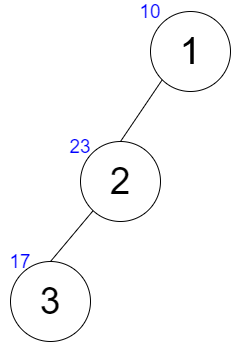# XOR TreeshefinCriterion 2021 Round 11
Limits 2s, 512 MB

You are given a tree with $N$ vertices. A tree is a connected undirected graph without cycles.

The tree has exactly one special vertex $X$. Each vertex $i$ has an integer number $A_i$ written on it. Let's denote a function $G(U)$ as the $\textbf{XOR}$ of all values $A_i$ written on the vertices on the simple path connecting vertex $U$ and the special vertex $X$. Let's denote another function $F(X)$ as the sum of all $G(i)$ where $X$ is the special vertex of the tree. More formally,
$F(X) = \sum\limits_{i=1}^{N} G(i),\space where\space X\space is\space the\space special\space vertex$

For each vertex $j$, you have to print the value of $F(j)$ treating vertex $j$ as the special vertex of the tree.

The simple path is the path that visits each vertex at most once. To know about $\textbf{XOR}$, you can read this.

## Input

The first line of the input will contain an integer $T(1\leq T\leq4\times10^4)$, the number of the test cases.

In each of the test cases, the first line will contain an integer $N(1\leq N\leq2\times10^5)$, the number of vertices of the tree. The next line will contain $N$ space-separated integers $A_1, A_2 ,...,A_N(0\leq A_i\leq 10^9)$, where $A_i$ the value written on vertex $i$. Each of the next $(N-1)$ lines will contain two integers $U$ and $V$ denoting there is an undirected edge between vertex $U$ and vertex $V$ $(1\leq U,V\leq N; U\neq V)$. It is guaranteed that the given edges form a tree.

The sum of $N$ over all the test cases will not be greater than $2\times10^{5}$.

## Output

For each test case, print $N$ space-separated integers $F(1),F(2),...,F(N)$ in a line, where $F(j)$ is the function described in the statement.

## Sample

InputOutput
2
3
10 23 17
1 2
2 3
4
13 56 9 35
2 4
2 3
1 2

51 58 35
148 185 136 102


Notes:

For test case $1$, the tree will be like this:The value of each vertex is written in blue. Let's see what happens when vertex $3$ is the special vertex in this tree.

$G(1) = 10\oplus23\oplus 17 = 12$
$G(2) = 23\oplus 17 = 6$
$G(3) = 17$

So, $F(3) = G(1) + G(2) + G(3) = 12+6+17 = 35$### Submit